Next: Two-Dimensional Jets Up: Two-Dimensional Incompressible Inviscid Flow Previous: Inviscid Flow Past a

# Inviscid Flow Over a Semi-Infinite Wedge

Consider the situation illustrated in Figure 5.12 in which an incompressible irrotational fluid flows over an impenetrable rigid wedge whose apex subtends an angle. Let the cross-section of the wedge in the-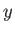plane be both-independent and symmetric about the-axis. Furthermore, let the apex of the wedge lie at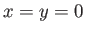. Finally, let the upstream flow a large distance from the wedge be parallel to the-axis.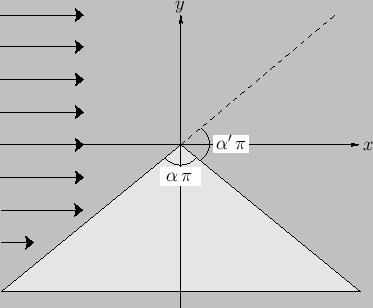Because the flow is two-dimensional, incompressible, and irrotational, it can be represented in terms of a stream function that satisfies Laplace's equation. The boundary conditions on the stream function are(5.130)

These boundary conditions ensure that the normal velocity at the surface of the wedge is zero. It is easily demonstrated that(5.131)

is a solution of Laplace's equation, (5.61). Moreover, this solution satisfies the boundary conditions provided that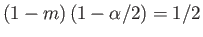, or(5.132)

where. Because the solutions to Laplace's equation are unique, we can again be sure that Equation (5.131) is the correct solution to the problem under investigation. According to this solution, the tangential velocity on the surface of the wedge is given by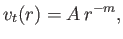(5.133)

where. Note that the tangential velocity, and hence the flow speed, is infinite at the apex of the wedge. However, this singularity in the flow can be eliminated by slightly rounding the apex. Figure 5.13 shows the streamlines of the flow for the case.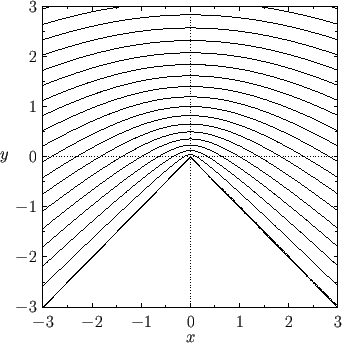Next: Two-Dimensional Jets Up: Two-Dimensional Incompressible Inviscid Flow Previous: Inviscid Flow Past a
Richard Fitzpatrick 2016-03-31